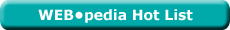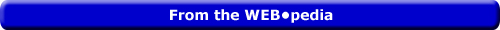Tuesday  June 28, 2022
 AmosWEB means Economics with a Touch of Whimsy!AD CURVE: The aggregate demand curve, which is a graphical representation of the relation between aggregate expenditures on real production and the price level, holding all ceteris paribus aggregate demand determinants constant. The aggregate demand, or AD, curve is one side of the graphical presentation of the aggregate market. The other side is occupied by the aggregate supply curve (which is actually two curves, the long-run aggregate supply curve and the short-run aggregate supply curve). The negative slope of the aggregate demand curve captures the inverse relation between aggregate expenditures on real production and the price level. This negative slope is attributable to the interest-rate effect, real-balance effect, and net-export effect.Most Viewed (Number) Visit the WEB*pediaLesson 22: Factor Supply | Unit 3: Factor Supply Page: 15 of 25

 Topic: Factor Cost Times Three <=PAGE BACK | PAGE NEXT=>

• A set of useful concepts in the analysis of factor markets:

• Total factor cost is the opportunity cost incurred when using a given factor of production to produce a good or service.
• Total factor cost is used as the starting point for calculating the other two related measures:

• Average factor cost is the total factor cost per unit of factor input, found by dividing total factor cost by the quantity of factor input.
• The third factor cost concept is marginal factor cost.

• Marginal factor cost is the change in total factor cost resulting from a change in the quantity of factor input, found by dividing the change in total factor cost by the change in quantity of factor input.
• Marginal factor cost indicates how a firm's total factor cost is affected by hiring one more or one fewer factor.

| | |INTERNATIONAL ECONOMICS

An economics field of study that applies both macroeconomic and microeconomic principles to international trade, which is the flow of trade among nations, and to international finance, which is the means of making payment for the exchange of goods among nations. International economics studies the economic interactions among the different nations that make up the global economy. Often this interaction is viewed in terms of the domestic economy and the foreign sector. The key economic principle underlying international economics is the law of comparative advantage.PINK FADFLY[What's This?] Today, you are likely to spend a great deal of time searching the newspaper want ads wanting to buy either a set of serrated steak knives, with durable plastic handles or a pair of blue silicon oven mitts. Be on the lookout for florescent light bulbs that hum folk songs from the sixties.Your Complete ScopeA U.S. dime has 118 groves around its edge, one fewer than a U.S. quarter."I do not believe in a fate that will fall on us no matter what we do. I do believe in a fate that will fall on us if we do nothing. "-- Ronald Reagan, 40th US presidentCSOCentral Statistical OfficeA PEDestrian's Guide Xtra CreditTell us what you think about AmosWEB. Like what you see? Have suggestions for improvements? Let us know. Click the User Feedback link.| | | | | | | | | | |
| | | |

Thanks for visiting AmosWEB